Effective ICSE Class 9 Maths Solutions S Chand Chapter 11 Rectilinear Figures Ex 11(C) can help bridge the gap between theory and application.

## S Chand Class 9 ICSE Maths Solutions Chapter 11 Rectilinear Figures Ex 11(C)

Question 1.
Construct a quadrilateral ABCD, when AB = 4 cm, BC = 6 cm, CD = DA = 5.2 cm and AC = 8 cm.
Solution:
Steps of constructions :
(i) Draw a line segment AB = 4 cm.
(ii) With centre A and radius 8 cm and with centre B and radius 6 cm, draw arcs which intersect each other at C.
(iii) Join AC and BC.
(iv) Again with centres A and C and radius 5.2 cm, draw two arcs intersecting each other at D.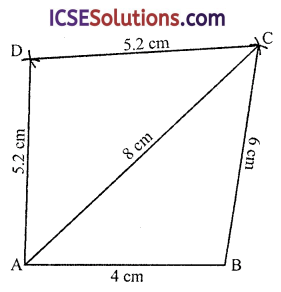Question 2.
Construct a quadrilateral PQRS, where PQ = 3.5 cm, QR = 5.5 cm, QS = 5.5 cm, PS = 4.5 cm and SR = 4.5 cm. Measure PR.
Solution:
Steps of constructions :
(i) Draw a line segment PQ = 3.5 cm.
(ii) With centre P and radius 4.5 cm and with centre Q and radius 5.5 cm, draw arcs intersecting each other at S.
(iii) Join RS and QS.
(iv) Again with center S and radius 4.5 and with centre Q and radius 5.5 cm, draw arcs intersecting each other at R.
(v) Join PR, QR and RS.
Then PQRS is the required quadrilateral on measuring PR = 7 cm.Question 3.
Construct a quadrilateral ABCD, when AB = 5 cm, BC = 4.2 cm, AD = 3.3 cm. AC = 5.5 cm and BD = 4.8 cm. Measure side CD.
Solution:
Steps of constructions :
(i) Draw a line segment AB = 5 cm.
(ii) With centre A and radius 5.5 cm, and centre B and radius 4.2 cm draw arcs intersecting each other at C.
(iii) Join AC and BC.
(iv) Again with centre A and radius 3.5 cm and centre B and radius 4.5 cm draw arcs intersecting each other at D.
(v) Join AD and BD and CD.
Then ABCD is the required quadrilateral on measuring CD, it is 2.5 cm.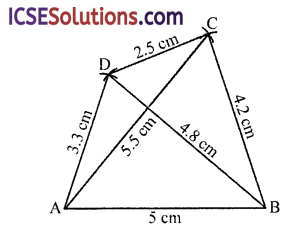Question 4.
Construct a quadrilateral ABCD, in which AB = BC = 4 cm, AD = CD = 6 cm and∠ABC= 120°.
Solution:
Steps of construction :
(i) Draw a line segment AB = 4 cm.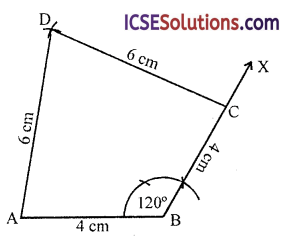(ii) At B, draw a ray BX making an angle of 120° and BC = 4 cm.
(iii) With centre A and radius 6 cm and centre C with radius 6 cm, draw arcs intersecting each other at D.
(iv) Join AB and CB.
Then ABCD is the required quadrilateral.

Question 5.
Construct a quadrilateral ABCD, in which AB = 4.6 cm, BC = 6.5 cm, CD = 6 cm, ∠B = 130° and ∠C = 75°.
Solution:
Steps of constructions :
(i) Draw a line segment BC = 6.5 cm.
(ii) At B, draw a ray BX making an angle of 130° and at C, a ray CY making another angle of 75°, and cut off CD = 6 cm and AB = 4.6 cm
Then ABCD is the required quadrilateral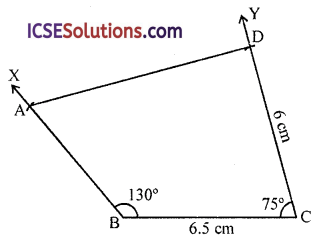Question 6.
Construct a quadrilateral PQRS, in which ∠Q= 45°, ∠R = 90°, QR = 5 cm, PQ = 9 cm and RS = 7 cm.
Solution:
Steps of constructions :
(i) Draw a line segment QR = 5 cm.
(ii) At Q, draw a ray QX making an angle of 45°.
(iii) At R, draw another ray making an angle of 90° and cut RS 7 cm.
(iv) Join PS.
Note : This figure is not possible to draw correctly.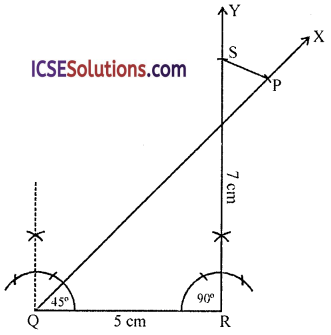Question 7.
Construct a quadrilateral ABCD, in which AB = 3.5 cm, BC = 6.5 cm.∠A = 75°, ∠B = 105° and ∠C = 120°.
Solution:
Steps of constructions :
(i) Draw a line segment AB = 3.5 cm.
(ii) At A, draw a ray AX making an angle of 55°.
(iii) At D, draw another ray BY making an angle of 105° and cut off BC = 6.5 cm.
(iv) At C, draw another ray CZ making an angle of 120° which intersects AX at D.
Then ABCD is the required quadrilateral.Question 8.
Construct a quadrilateral PQRS where PQ = 3.8 cm, QR = 6.8 cm, ∠P = ∠R = 105° and ∠S = 75°.
Solution:
Steps of constructions :
∠Q = 360° – (∠P + ∠R + ∠S) = 360° – (105° + 105° + 75°) = 360° – 285° = 75°
(i) Draw a line segment QR = 6.8 cm.
(ii) At A, draw a ray QX making an angle of 75° and cut off PQ = 3.8 cm.
(iii) At C draw another ray making an angle of 105° and at R, draw a ray making an angle of 105° meeting each other at S.
Then PQRS is the required quadrilateral.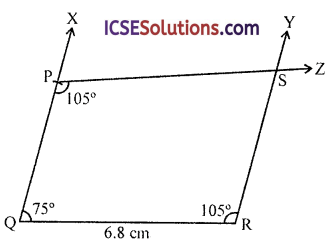Question 9.
Using ruler and compasses only construct the quadrilateral ABCD having given AB = 5 cm, BC = 2.5 cm, CD = 6 cm, ∠BAD = 90° and the diagonal AC = 5.5 cm.
Solution:
Steps of constructions :
(i) Draw a line segment AB = 5 cm.
(ii) With centre A and radius 5.5 cm and with centre B and radius 2.5 cm draw arcs intersecting each other at C.
(iii) Join AC and BC.
(iv) At A draw a ray AX making an angle of 90°.
(v) With centre C and radius 6 cm, draw an arc which intersects AX at D.Question 10.
Draw a quadrilateral ABCD with AB = 6 cm, BC = 4 cm, CD = 4 cm and∠ABC = ∠BCD = 90°. (ICSE)
Solution:
Steps of constructions :
(i) Draw a line segment BC = 4 cm.
(ii) At B and C, draw rays BX and CY making 90° angle each.
(iii) From CY cut off CD = 4 cm and from AX, cut off BA = 6 cm.